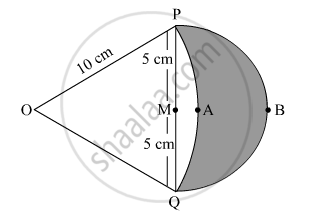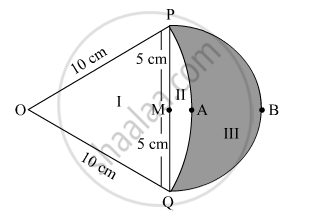Share

# In Fig. 7, are shown two arcs PAQ and PBQ. Arc PAQ is a part of circle with centre O and radius OP while arc PBQ is a semi-circle drawn on PQ ad diameter with centre M. If OP = PQ = 10 cm show that area of shaded region is - CBSE Class 10 - Mathematics

ConceptProblems Based on Areas and Perimeter Or Circumference of Circle, Sector and Segment of a Circle

#### Question

In Fig. 7, are shown two arcs PAQ and PBQ. Arc PAQ is a part of circle with centre O and radius OP while arc PBQ is a semi-circle drawn on PQ ad diameter with centre M. If OP = PQ = 10 cm show that area of shaded region is 25(sqrt3-pi/6)cm^2.#### Solution

Given: OP = OQ = 10 cm

It is known that tangents drawn from an external point to a circle are equal in length.

So,
OP = OQ = 10 cmTherefore, ABC is an equilateral triangle.

POQ=60°

Now

Area of part II = Area of the sector Area of the equilateral triangle POQ

=(/_POQ)/360^@xxpir^2-sqrt3/4xx(10)^2

=60^@/360^@xxpi(10^2)-sqrt3/4xx(10)^2

=100(pi/6-sqrt3/4)

Area of the semicircle on diameter PQ = Area of part II + Area of part III

1/2xxpi(5)^2=25/2pi

Area of the shaded region (part III)

=25/2pi-100(pi/6-sqrt3/4)

=25/2pi-100/6pi+25sqrt3

=25sqrt3-25/6pi

=25(sqrt3-pi/6)

Hence proved.

Is there an error in this question or solution?

#### Video TutorialsVIEW ALL 

Solution In Fig. 7, are shown two arcs PAQ and PBQ. Arc PAQ is a part of circle with centre O and radius OP while arc PBQ is a semi-circle drawn on PQ ad diameter with centre M. If OP = PQ = 10 cm show that area of shaded region is Concept: Problems Based on Areas and Perimeter Or Circumference of Circle, Sector and Segment of a Circle.
S